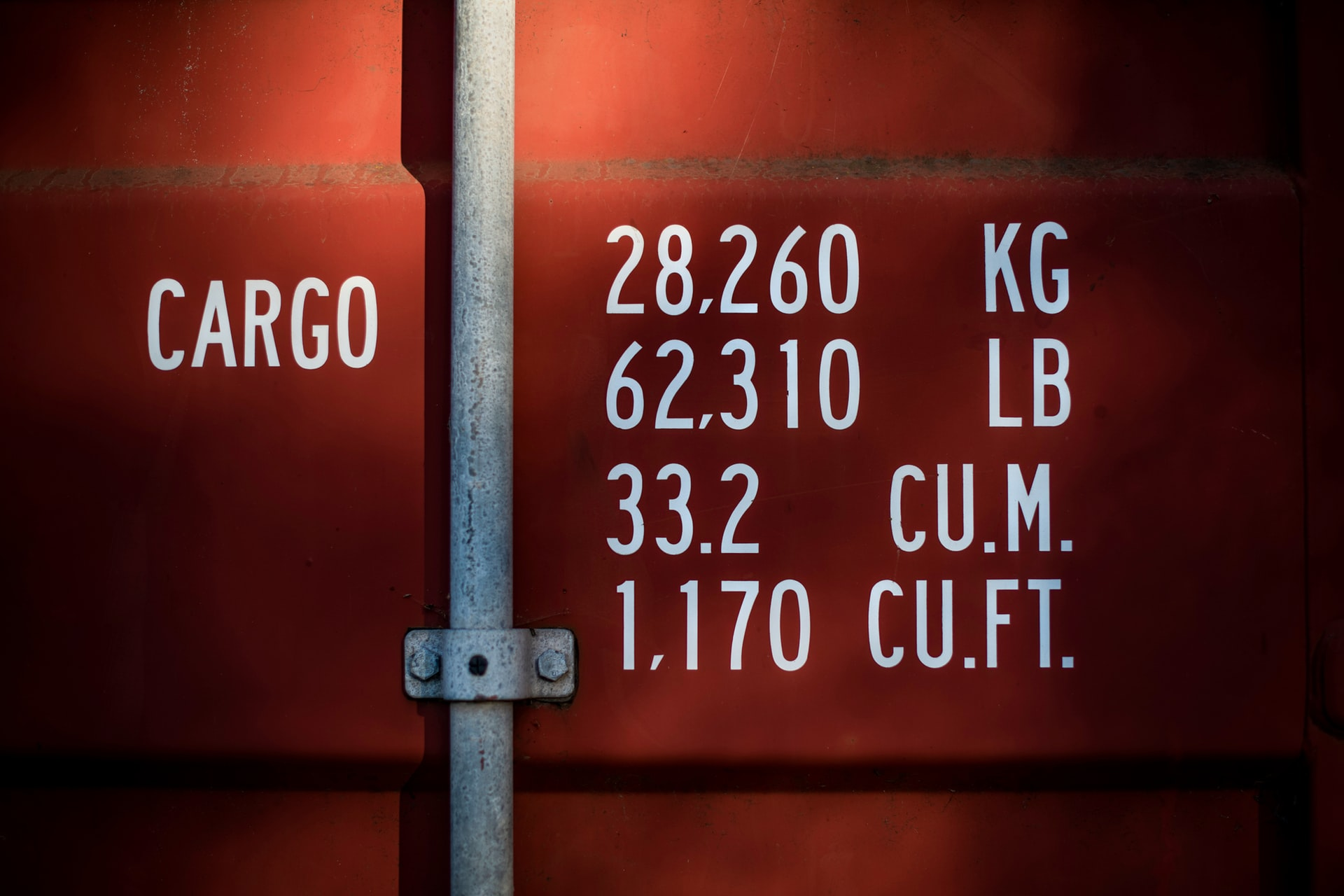How to calculate your ocean freight volume is important so that you can decide whether you want to pay for a Less-than-Container Load (LCL) or Full Container Load (FCL).

How to calculate your ocean freight volume is important so that you can decide whether you want to pay for a Less-than-Container Load (LCL) or Full Container Load (FCL).

#### How is it calculated then?

There are a number of different ways you can calculate but I will show the below as an example:

• First convert your length, width and height to a part of a metre, usually up to two decimal places.
• So, 40 X 40 X 30 cms becomes 0.40 X 0.40 X 0.30.
• Multiple each by the number of cartons you have.
• So, 50 cartons would be 50 X 0.40 X 0.40 X 0.30 = 2.40CBM

You need to decide if you will need to go with LCL or FCL. Once you know what you have to ship you need to discuss with a freight forwarder.  The forwarder will calculate the weight of the cargo and cubic volume (CBM) of cargo called the ‘measure’. Compare these on the basis that 1CBM = 1000kgs and whichever is greater of the two the forwarder will calculate the cost.

#### Further information on Wikipedia:

https://en.wikipedia.org/wiki/Dimensional_weight

#### What other choices do I have?

There will still be different factors to consider aside from how much you are sending; alternative modes of transport may be preferable.   It can work out overall cheaper (and quicker) to look at an economy air option or a road option.

One of the major differences is with smaller cargo the freight rate may be less but the associated costs would be higher than air.  I’ll cover this in another post as there are a number of details which need further explanation.  It can be a bit complicated and its important that shippers or importers understand everything to expect.

For ocean there aren’t many restrictions on the types of goods lines can send this way but other modes may be limited.  That may make ocean the buyer’s only choice if that’s the case.

So a simple visualisation on how to calculate your ocean freight volume is below:

Want to understand how to calculate airfreight volume as well?